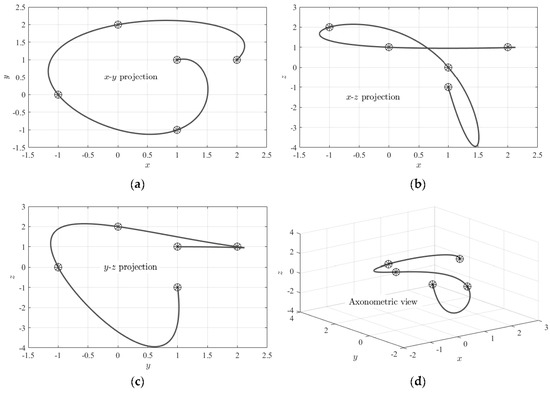Next Article in Journal
Invariant Solutions for a Class of Perturbed Nonlinear Wave Equations
Previous Article in Journal
A Constructive Method for Standard Borel Fixed Submodules with Given Extremal Betti Numbers
Open AccessArticle

The Theory of Connections: Connecting Points†

byDaniele MortariAerospace Engineering, Texas A & M University, College Station, TX 77843-3141, USA
This paper is an extended version of our paper published in Mortari, D. The Theory of Connections. Part 1: Connecting Points, AAS 17-255, 2017 AAS/AIAA Space Flight Mechanics Meeting Conference, San Antonio, TX, USA, 5–9 February 2017; (dedicated to John Lee Junkins).
Mathematics 2017, 5(4), 57; https://doi.org/10.3390/math5040057
Received: 30 July 2017 / Revised: 17 September 2017 / Accepted: 24 October 2017 / Published: 1 November 2017
This study introduces a procedure to obtain all interpolating functions, $y = f ( x )$ , subject to linear constraints on the function and its derivatives defined at specified values. The paper first shows how to express these interpolating functions passing through a single point in three distinct ways: linear, additive, and rational. Then, using the additive formalism, interpolating functions with linear constraints on one, two, and n points are introduced as well as those satisfying relative constraints. In particular, for expressions passing through n points, a generalization of the Waring’s interpolation form is introduced. An alternative approach to derive additive constraint interpolating expressions is introduced requiring the inversion of a matrix with dimensions equally the number of constraints. Finally, continuous and discontinuous interpolating periodic functions passing through a set of points with specified periods are provided. This theory has already been applied to obtain least-squares solutions of initial and boundary value problems applied to nonhomogeneous linear differential equations with nonconstant coefficients. View Full-Text
Keywords:
Show FiguresFigure 1

MDPI and ACS Style

Mortari, D. The Theory of Connections: Connecting Points. Mathematics 2017, 5, 57.

Show more citation formats Show less citations formats
Note that from the first issue of 2016, MDPI journals use article numbers instead of page numbers. See further details here.

1